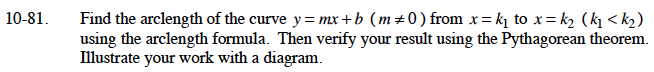### Home > CALC > Chapter 10 > Lesson 10.1.8 > Problem10-81

10-81.$=\left.x\sqrt{1+m^2}\right|_{k_1}^{k_2}=?$

If you were to create a slope triangle for the points (k1, k1m + b) and (k2, k2m + b), what would be the length of each leg of the slope triangle?

$\sqrt{(k_2-k_1)^2+((k_2m+b)-(k_1m+b))^2}$